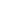To the editors:

The solution to the hen problem (Straight Dope, June 29), can be greatly simplified, and the “riddle” Cecil refers to resolved by redefining the problem as follows: A giant and his wife share three hens, equally between them. They find two eggs in the chicken coop each morning and each gets one egg to each. That is, each gets one egg a day, or one and one-half eggs in one and one-half days, or, if you like, pi eggs in pi days, or the square root of two eggs in the square root of two days, or the six eggs in six days we are looking for, from his or her one and one-half hens. The correct answer now is even more obvious than Marilyn’s already “too obvious” incorrect answer, and is gotten without Cecil’s “algebra.”

Another solution, which attacks the “riddle” aspect from a different angle, is to divide the six-day deadline into 1.5-day periods, and multiply the result, four, by each 1.5-hen team’s output during that period (1.5 eggs). The result is the half-dozen eggs we are hoping for.

The “riddle” in this case refers to the fact that dividing the six-day cycle by the period in question and then multiplying by each work group’s output during that period is obviously going to yield 6 when the output equals the period; in other words, whenever the output per work group equals the period in which that output is achieved, the number of hens required to produce x eggs in x days is equal to the given size of the work group.

Hence, if you, the Reader, find that if you have to increase your staff by 50% in order to put out one and one-half issues every one and one-half weeks, then you will need that same staff to put out six issues every six weeks.